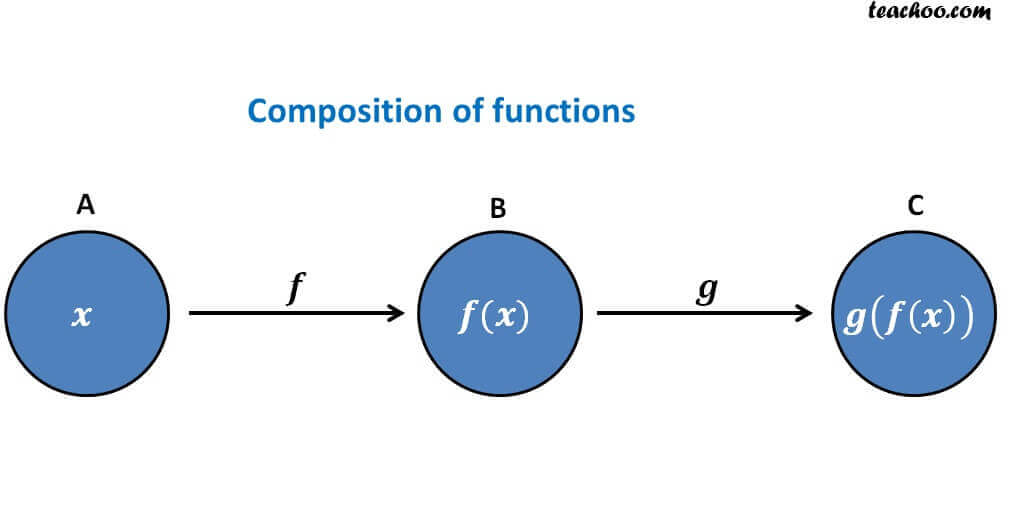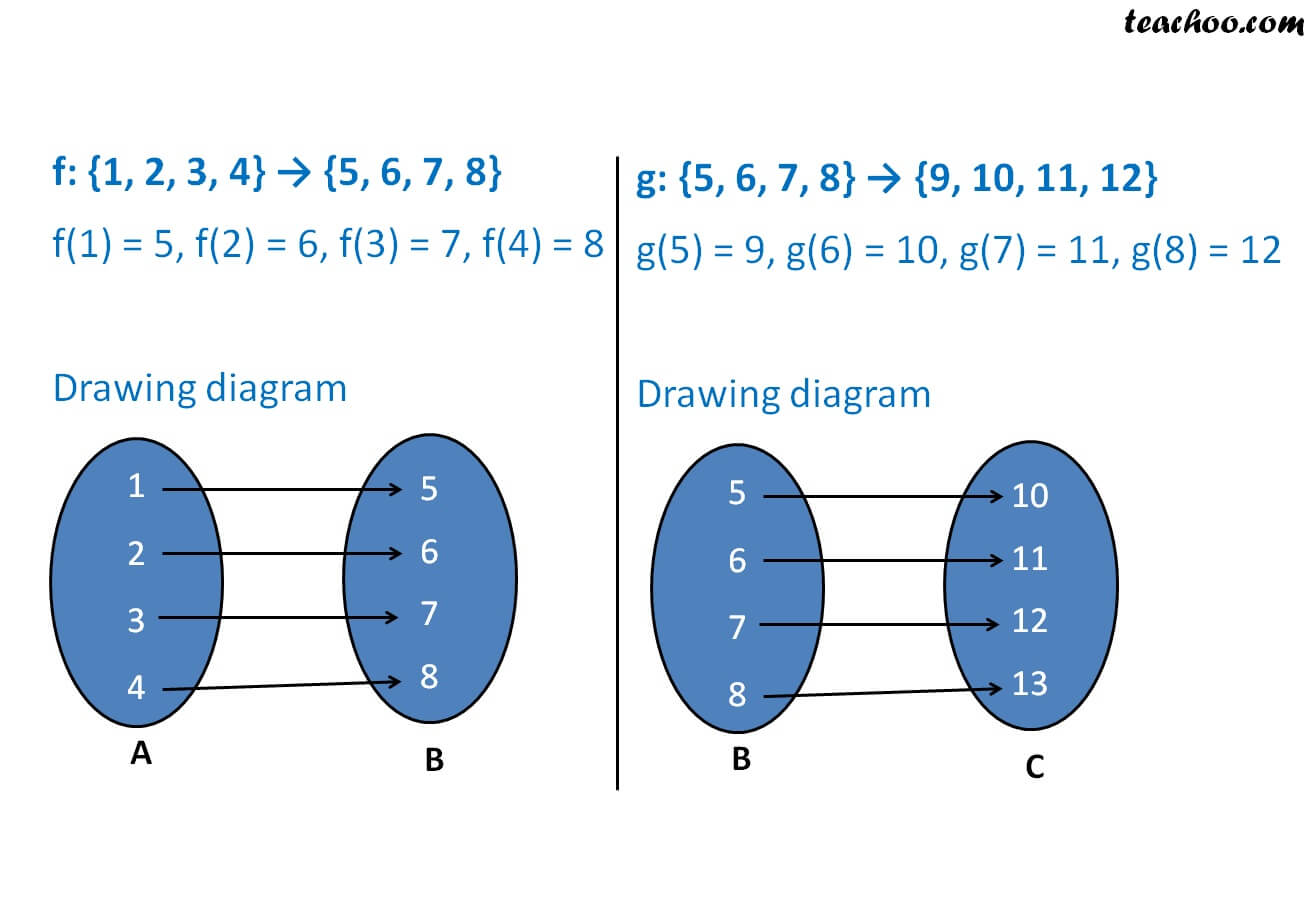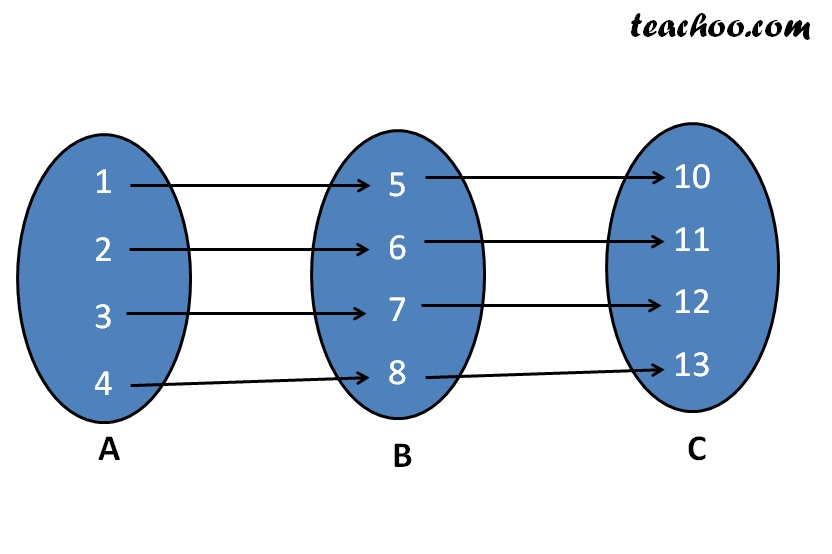Composite functions

Chapter 1 Class 12 Relation and Functions
Concept wise

If f: A → B, g: B → C

Then

gof : A → C

gof = g(f(x))

Here, gof is formed by the composition of functions f and g.In gof :

• Value of x is coming from set A
• Value of function gof will be from set C

Let us take an example

Let f: {1, 2, 3, 4} → {5, 6, 7, 8}

f(1) = 5, f(2) = 6, f(3) = 7, f(4) = 8

and

g: {5, 6, 7, 8} → {9, 10, 11, 12}

g(5) = 9, g(6) = 10, g(7) = 11, g(8) = 12

Find gofgof will begof (1) = 10

gof (2) = 11

gof (3) = 12

gof (4) = 13

Let’s take another example

f: R R , g: R R

f(x) = sin x , g(x) = x 3

Find fog and gof

f(x) = sin x

f(g(x)) = sin g(x)

f og (x) = sin (x 3 )

g(x) = x 3

g(f(x)) = f(x) 3

go f (x) = sin 3 x

Note that go f f og .

Learn in your speed, with individual attention - Teachoo Maths 1-on-1 Class